# Short Notes: Inverse Trigonometric FunctionsA function  f : A → B is said to be invertible if f is bijective and their inverse function   f-1 : B → A is defined by

f-1(y) = x if f(x) = y , x ∈ A, y ∈  B

We know that trigonometric functions are periodic functions, and hence, in general, all trigonometric functions are not bijections. Consequently, their inverse do not exist. However, if we restrict their domain and co-domains, they can be made bijections and we can obtain their inverse. The graphs of trigonometric functions gave a fare idea of the domain where they are invertible.

# Graphs of Functions and their Inverse

Sine function and its inverse: Consider the function    f : R → R given by . f(x)  =  sinx. It is a many-one into function as it attains same value at infinitely many points and its range [– 1, 1] is not same as its co-domain. But we know that any function can be made an onto function by replacing its co-domain by its range.

Therefore, f : R → [−1,1]is a many-one onto function. In order to make f a one-one function, we will have to restrict its domain in such a way that in that domain there is no turn in the graph of the function and the function takes every value between –1 and 1. It is evident from the graph of  f(x) = sin(x) that if we take the domain as [-π/2, π/2] then f(x) becomes one-one.

Thus,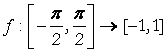given by f(x) = sin(x) is a bijection and hence it is invertible.

Thus,  sin-1x is a function with domain [– 1, 1] and rangesuch that

sin-1x  = θ = ⇔ sinθ =x

The graph of the function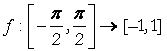given by  f(x) = sinx as shown in fig. 2.1. In order to obtain the graph of sin-1x   we interchange and y axes as shown in the following figures.Fig. 2.1                                                                     Fig. 2.2

Cosine function and its inverse: The function  f : [0,π] → [−1,1] given by  f(x) = cosx is a bijection. Therefore, cos-1x  is a function with domain [–1, 1] and range  [0,π] the graph of cos-1x  is obtained from the graph of cosine function by interchanging x and y axes as shown in figures 2.3 and 2.4.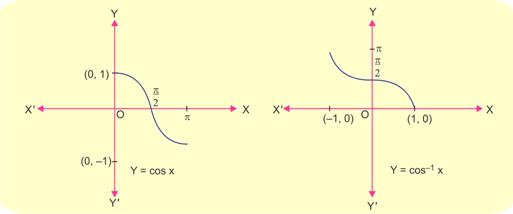Fig. 2.3 Fig. 2.4

Tangent Function and its inverse: The function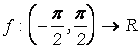given by f(x) = tanx is a bijection and hence it is invertible. The domain of definition of the function tan-1x is the whole set R of real numbers and range is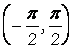. The graph of tan-1x is obtained from the graph of function tan x by interchanging x and y axes as shown in figures.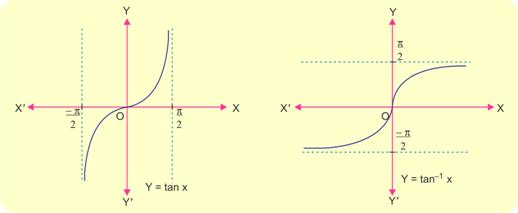Cotangent function and its inverse : The function f : [0,π] → R given by  f(x ) = cotx is a bijection and hence invertible. Therefore,  cot-1x : R → (0,π) exists. The graphs of  y = cotx and y = cot-1x are shown in figures. The domain of function cot-1x is R (set of all real numbers and its range is (0,π) .Secant Function and its Inverse: The functiongiven by  f(x) = secx is a bijection and hence invertible. Therefore, sec-1x is a function with domain  [−∞,-1] ∪[1,∞]and range [0,π]-{π/2} . The graph of y= secx and  y = sec-1x are shown in figures.Cosecant and its inverse function : The function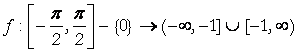defined by   f(x) = cosec x is a bijective function. Hence it is invertible. Therefore,   cosec-1x is a function with domain  [−∞,−1] ∪[1,∞] and range [-π/2, π/2] – {0}.

The graphs of  y= cosec x , y = cosec-1x  are shown in figures.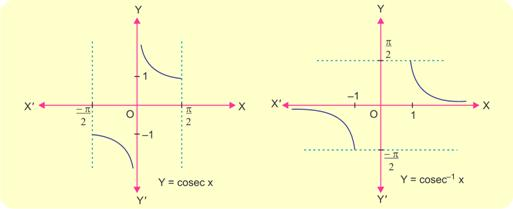# General and Principal Values of Inverse trigonometric Functions

We know that for  −1 ≤ y ≤ 1 there are infinitely many angles which satisfy the equation sinx =y . This infinite number of angles is symbolically denoted by sin-1y . This infinite set of values is called the set of general values and the value that lies in the interval [-π/2, π/2] is called principal value.

In compact form, we may write it as:Similarly, the definitions of the other inverse trigonometrical functions can be given. We tabulate definition with principal and general values as follows:## Points to be Remembered

1.and so on.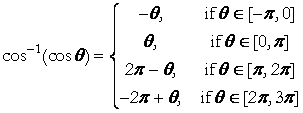and so on.and so on.

2.

sin(sin-1x) = x for all x∈ [−1,1]

cos(cos-1x) = x for all x∈ [−1,1]

tan(tan-1x) = x for all x∈ R

cot(cot-1x) = x for all x∈ R

sec(sec-1x) = x for all x∈ (−∞,−1]∪[1,∞)

cosec(cosec-1x) = x for all x∈ (−∞,−1]∪[1,∞)

3. sin-1(−x) = − sin-1x, for all x∈ [−1,1]

cos-1(−x) = π − cos-1x, for all x∈ [−1,1]

tan-1(−x) = − tan-1x, for all x∈ R

cot-1(−x) = π − cot-1x, for all x∈ R

sec-1(−x) = π − sec-1x, for all x∈ (−∞,−1]∪[1,∞)

cosec-1(−x) = − cosec-1x  for all x∈ (−∞,−1]∪[1,∞)

4.5.6.where  x1,x2,x3,……….xn  ∈  R and Sk denotes the sum of the products of  x1,x2,x3,……….xn  taken k at a time.

.ie., S1 = x1 + x2 + x3 +,……….+ xn.

S2 = x1x2 + x1x3 + x1x4 +,……….+ x1xn + x2x3 + x2x4 + …… + x2xn, etc

7.8.9.10.In order to simplify trigonometrical expression involving inverse trigonometrical functions, following substitutions are very useful:

 Expression Substituion a2  + x2 x=a tanθ or x=a cotθ a2  − x2 x=a sinθ or x=a cosθ x2  − a2 x=a sec θ or x=a cosec θ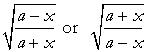x=a cos2θ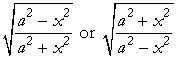x2  = a2 cos2θ